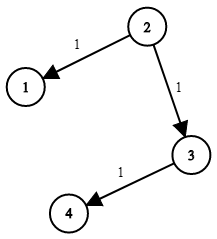# 743. Network Delay Time

Medium
You are given a network of `n` nodes, labeled from `1` to `n`. You are also given `times`, a list of travel times as directed edges `times[i] = (ui, vi, wi)`, where `ui` is the source node, `vi` is the target node, and `wi` is the time it takes for a signal to travel from source to target.
We will send a signal from a given node `k`. Return the minimum time it takes for all the `n` nodes to receive the signal. If it is impossible for all the `n` nodes to receive the signal, return `-1`.
Example 1:Input: times = [[2,1,1],[2,3,1],[3,4,1]], n = 4, k = 2
Output: 2
Example 2:
Input: times = [[1,2,1]], n = 2, k = 1
Output: 1
Example 3:
Input: times = [[1,2,1]], n = 2, k = 2
Output: -1
Constraints:
• `1 <= k <= n <= 100`
• `1 <= times.length <= 6000`
• `times[i].length == 3`
• `1 <= ui, vi <= n`
• `ui != vi`
• `0 <= wi <= 100`
• All the pairs `(ui, vi)` are unique. (i.e., no multiple edges.)

### 解題

func networkDelayTime(times [][]int, n int, k int) int {
// 從 source 出發，求到全部節點的最短路徑
// 有節點不能到達，回傳 -1
dist := make([]int, n)
for i := 0 ; i < n ; i++ {
dist[i] = math.MaxInt32
}
dist[k - 1] = 0 //source
for i := 1; i < n ; i++ {
for _, time := range times {
dist[time - 1] = min(dist[time - 1], dist[time - 1] + time)
}
}
ans := -1
for _, dis := range dist {
if dis == math.MaxInt32 { //有節點不能到達
return -1
}
if dis > ans {
ans = dis
}
}
return ans
}
func min(a, b int) int {
if a < b { return a }
return b
}